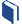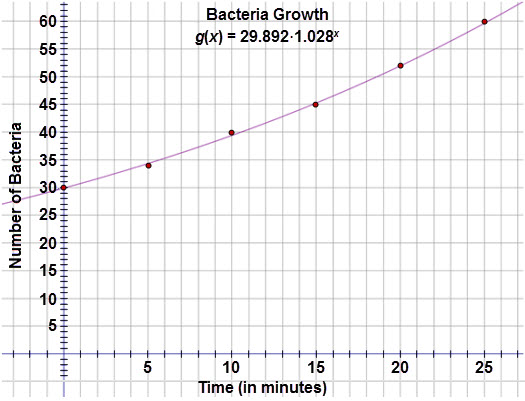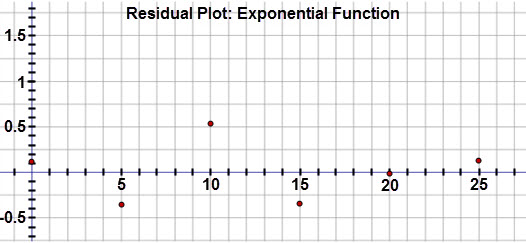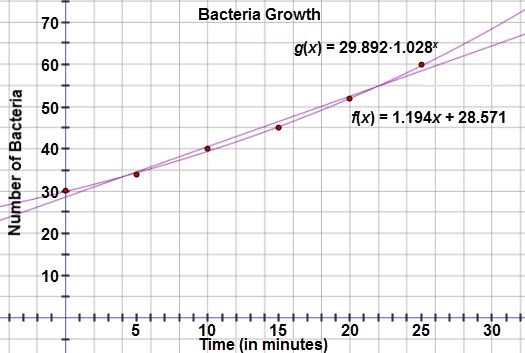# A Deeper Look at Exponential Functions

###Resources for this lesson:

> Glossary> Calculator Resources> Teacher Resources: Instructional Notes## Test and Confirm

Examine the exponential curve of best fit on the scatter plot:The graph appears to pass through most of the points.  Now examine the residual plot:Inspection of the residuals reveals that these values are very small and in a random pattern, confirming that this is the best fit for the data set.

Let’s examine each model on the scatter plot.  Notice that exponential curve almost touches each of the data points.  The linear model has greater gaps between the curve and some of the data points.When you compare the residual plots side by side, notice that the residuals for the exponential model are much smaller than the linear model. This provides further evidence that the exponential model is in fact the better fit.